Showing first {{hits.length}} results of {{hits_total}} for {{searchQueryText}}{{hits.length}} results for {{searchQueryText}}

No Search Results

The use of superscripts and subscripts is very common in mathematical expressions involving exponents, indexes, and in some special operators. This article explains how to write superscripts and subscripts in simple expressions, integrals, summations, et cetera. Contents 1 Introduction 2 More detailed examples 3 Operators using subscripts and superscripts 4 Reference guide 4.1 Further reading Introduction Definite integrals are some of the most common mathematical expressions, let's see an example: $\int\limits_0^1 x^2 + y^2 \ dx$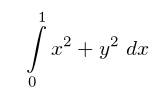In LaTeX, subscripts and superscripts are written using the symbols ^ and _, in this case the x and y exponents where written using these codes. The codes can also be used in some types of mathematical symbols, in the integral included in the example the _ is used to set the lower bound and the ^ for the upper bound. The command \limits changes the way the limits are displayed in the integral, if not present the limits would be next to the integral symbol instead of being on top and bottom. (see the reference guide)   Open an example in Overleaf More detailed examples The symbols _ and ^ can also be combined in the same expression, for example: $a_1^2 + a_2^2 = a_3^2$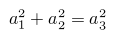If the expression contains long superscripts or subscripts, these need to be collected in braces, as LaTeX normally applies the mathematical commands ^ and _ only to the following character: $x^{2 \alpha} - 1 = y_{ij} + y_{ij}$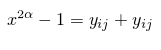Subscripts and superscripts can be nested and combined in various ways. When nesting subscripts/superscripts, however, remember that each command must refer to a single element; this can be a single letter or number, as in the examples above, or a more complex mathematical expression collected in braces or brackets. For example: $(a^n)^{r+s} = a^{nr+ns}$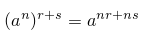Open an example in Overleaf Operators using subscripts and superscripts Some mathematical operators may require subscripts and superscripts. The most frequent cases are those of the integral \int (check the introduction) and the summation (\sum) operators, whose bounds are typeset precisely with subscripts and superscripts. $\sum_{i=1}^{\infty} \frac{1}{n^s} = \prod_p \frac{1}{1 - p^{-s}}$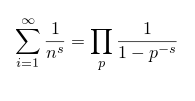For other frequently used operators that require subscripts/superscripts check the reference guide.   Open an example in Overleaf Reference guide Additional examples and operators LaTeX markup Renders as a_{n_i} $a_{n_{i}}$\int_{i=1}^n $\int _{i=1}^{n}$\sum_{i=1}^{\infty} $\sum _{i=1}^{\infty }$\prod_{i=1}^n $\prod _{i=1}^{n}$\cup_{i=1}^n $\cup _{i=1}^{n}$\cap_{i=1}^n $\cap _{i=1}^{n}$\oint_{i=1}^n $\oint _{i=1}^{n}$\coprod_{i=1}^n $\coprod _{i=1}^{n}$There are also a bigcup and bigcap commands similar to cup and cap but larger for larger expressions.   Open an example in Overleaf Further reading For more information see List of Greek letters and math symbols Operators Integrals, sums and limits Mathematical fonts Brackets and Parentheses The Great, Big list of LaTeX Symbols (a full list of available mathematical symbols in the amssymb package) The not so short introduction to LaTeX2ε Aligning equations with amsmath